# Matrices & Determinants Questions and Answers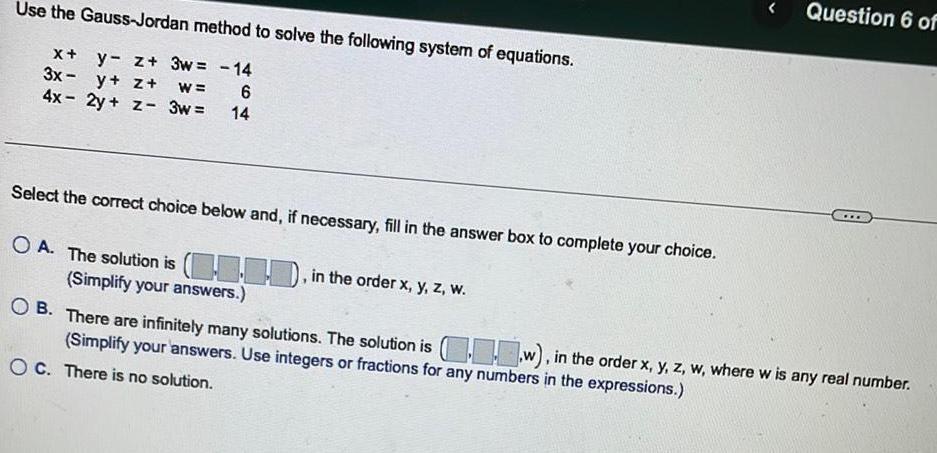Algebra
Matrices & Determinants
Use the Gauss-Jordan method to solve the following system of equations. x+y- z+ 3w= -14 3x-y+z+w = 6 4x - 2y + z- 3w= 14 Select the correct choice below and, if necessary, fill in the answer box to complete your choice. A. The solution is ..., in the order x, y, z, w. B. There are infinitely many solutions. The solution is (...w), in the order x, y, z, w, where w is any real number. (Simplify your answers. Use integers or fractions for any numbers in the expressions.) C. There is no solution.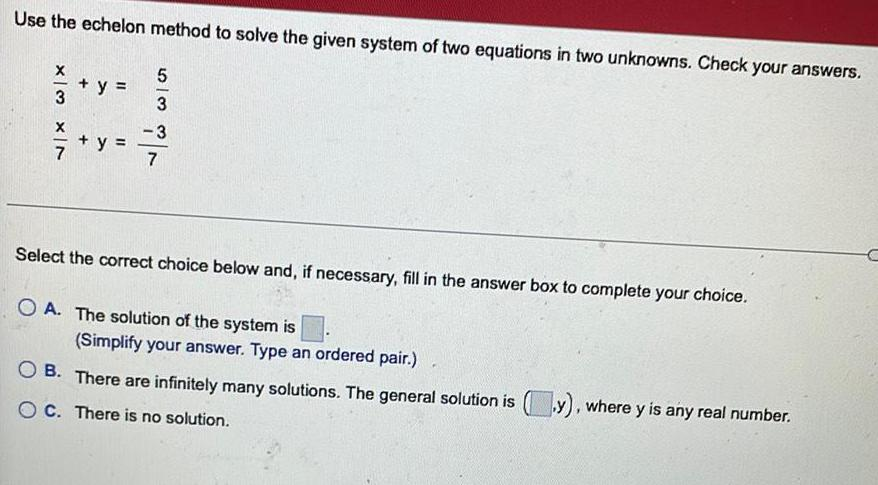Algebra
Matrices & Determinants
Use the echelon method to solve the given system of two equations in two unknowns. Check your answers. X/3 + y = 5/3 x/7 + y =-3/7 Select the correct choice below and, if necessary, fill in the answer box to complete your choice. A. The solution of the system is (Simplify your answer. Type an ordered pair.) B. There are infinitely many solutions. The general solution is y), where y is any real number. OC. There is no solution.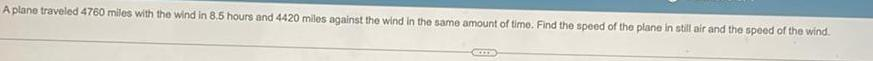Algebra
Matrices & Determinants
A plane traveled 4760 miles with the wind in 8.5 hours and 4420 miles against the wind in the same amount of time. Find the speed of the plane in still air and the speed of the wind.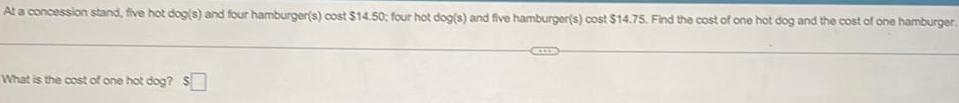Algebra
Matrices & Determinants
At a concession stand, five hot dog(s) and four hamburger(s) cost \$14.50; four hot dog(s) and five hamburger(s) cost \$14.75. Find the cost of one hot dog and the cost of one hamburger. What is the cost of one hot dog?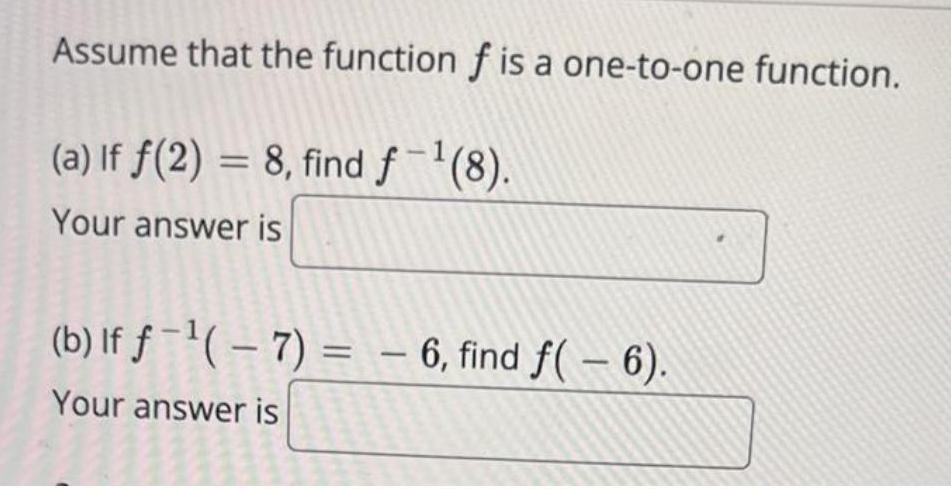Algebra
Matrices & Determinants
Assume that the function f is a one-to-one function. (a) If f(2)= 8, find f-¹(8). Your answer is (b) If f¹(-7)= -6, find f(- 6). Your answer is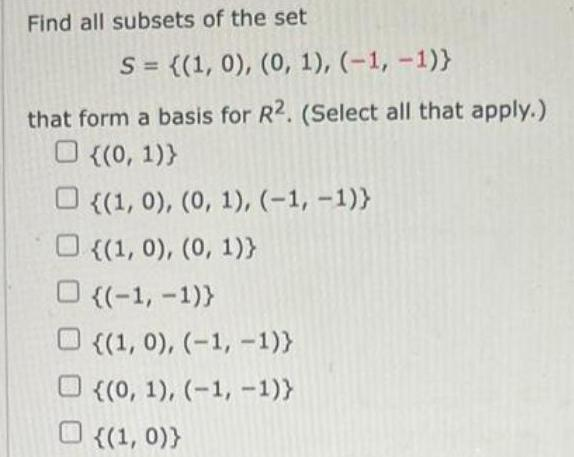Algebra
Matrices & Determinants
Find all subsets of the set S = {(1, 0), (0, 1), (-1,-1)} that form a basis for R2. (Select all that apply.) {(0, 1)) {(1, 0), (0, 1), (-1, -1)} {(1, 0), (0, 1)} ((-1,-1)} {(1, 0), (-1, -1)} {(0, 1), (-1, -1)} {(1, 0))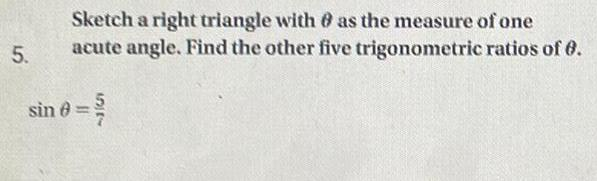Algebra
Matrices & Determinants
Sketch a right triangle with θ as the measure of one acute angle. Find the other five trigonometric ratios of θ. sin θ = 5/7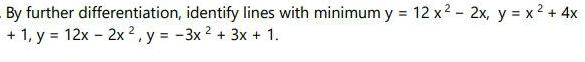Algebra
Matrices & Determinants
By further differentiation, identify lines with minimum y = 12 x² - 2x, y = x² + 4x + 1, y = 12x - 2x2, y = -3x² + 3x + 1.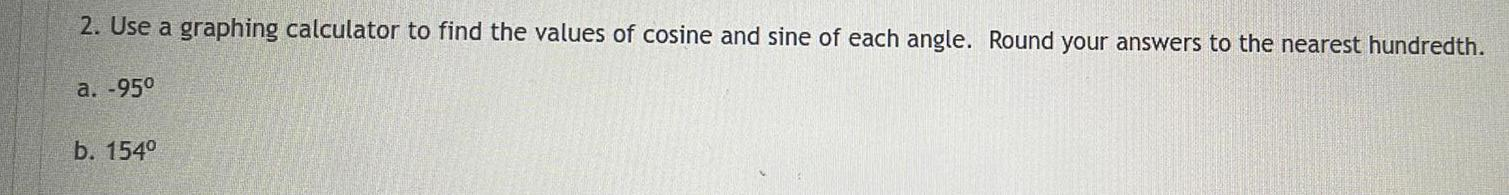Algebra
Matrices & Determinants
Use a graphing calculator to find the values of cosine and sine of each angle. Round your answers to the nearest hundredth. a. -95⁰ b. 154⁰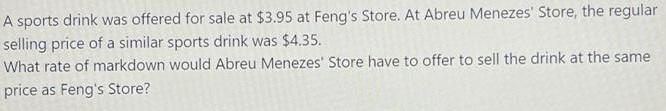Algebra
Matrices & Determinants
A sports drink was offered for sale at \$3.95 at Feng's Store. At Abreu Menezes' Store, the regular selling price of a similar sports drink was \$4.35. What rate of markdown would Abreu Menezes' Store have to offer to sell the drink at the same price as Feng's Store?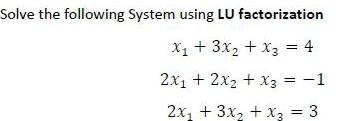Algebra
Matrices & Determinants
Solve the following System using LU factorization x₁ + 3x₂ + x3 = 4 2x₁ + 2x₂ + x3 = -1 2x₁ + 3x₂ + x3 = 3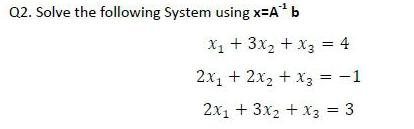Algebra
Matrices & Determinants
Solve the following System using x=A¹ b X₁ + 3x₂ + x3 = 4 2x₁ + 2x₂ + x3 = -1 2x₁ + 3x2 + x3 = 3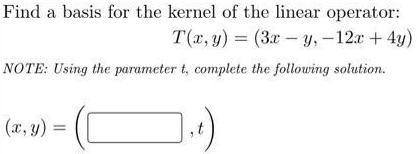Algebra
Matrices & Determinants
Find a basis for the kernel of the linear operator: T(x, y) = (3x-y, -12x + 4y) NOTE: Using the parameter t, complete the following solution.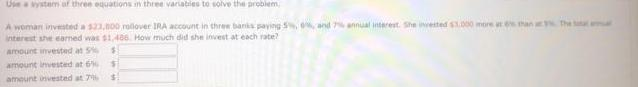Algebra
Matrices & Determinants
Use a system of three equations in three variaties to solve the problem, A woman invested a \$23,000 rollover IRA account in three banks paying 5%, 0%, and 7% annual interest. She invested \$1.000 more at 6s than a The Smal interest she earned was 11,486. How much did she invest at each rate? amount invested at 5% \$ amount invested at 6% amount invested at 7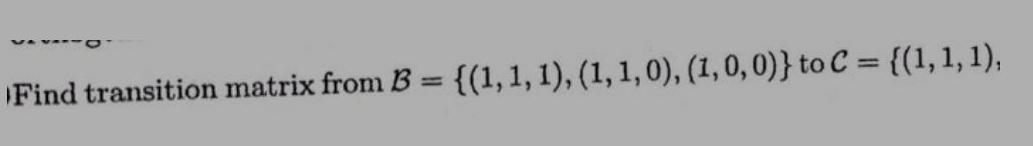Algebra
Matrices & Determinants
Find transition matrix from B = {(1, 1, 1), (1, 1, 0), (1, 0, 0)} to C = {(1, 1, 1),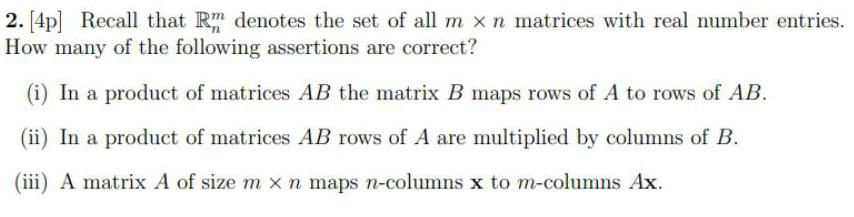Algebra
Matrices & Determinants
Recall that Rm denotes the set of all m x n matrices with real number entries. How many of the following assertions are correct? (i) In a product of matrices AB the matrix B maps rows of A to rows of AB. (ii) In a product of matrices AB rows of A are multiplied by columns of B. (iii) A matrix A of size m x n maps n-columns x to m-columns Ax.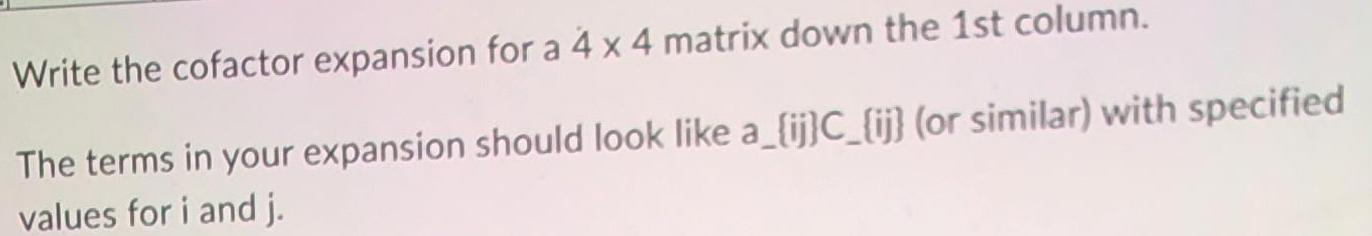Algebra
Matrices & Determinants
Write the cofactor expansion for a 4 x 4 matrix down the 1st column. The terms in your expansion should look like a_{ij}C_{ij} (or similar) with specified values for i and j.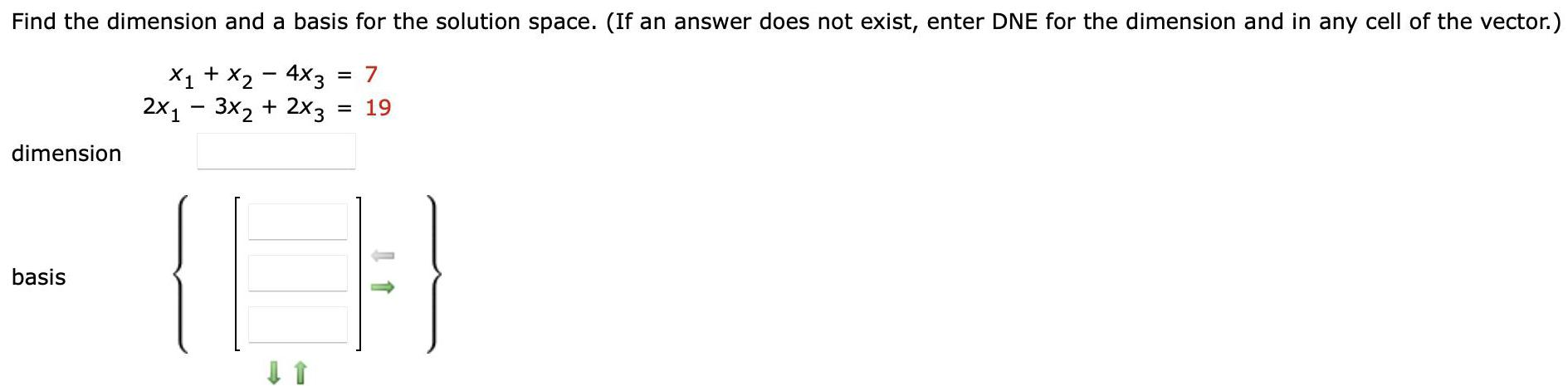Algebra
Matrices & Determinants
Find the dimension and a basis for the solution space. (If an answer does not exist, enter DNE for the dimension and in any cell of the vector.) x₁ + x2 - 4x3 = 7 2x13x₂ + 2x3 = 19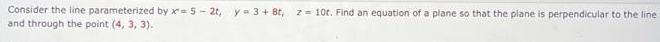Algebra
Matrices & Determinants
Consider the line parameterized by x=5-2t, y = 3+ 8t, z= 10t. Find an equation of a plane so that the plane is perpendicular to the line and through the point (4, 3, 3).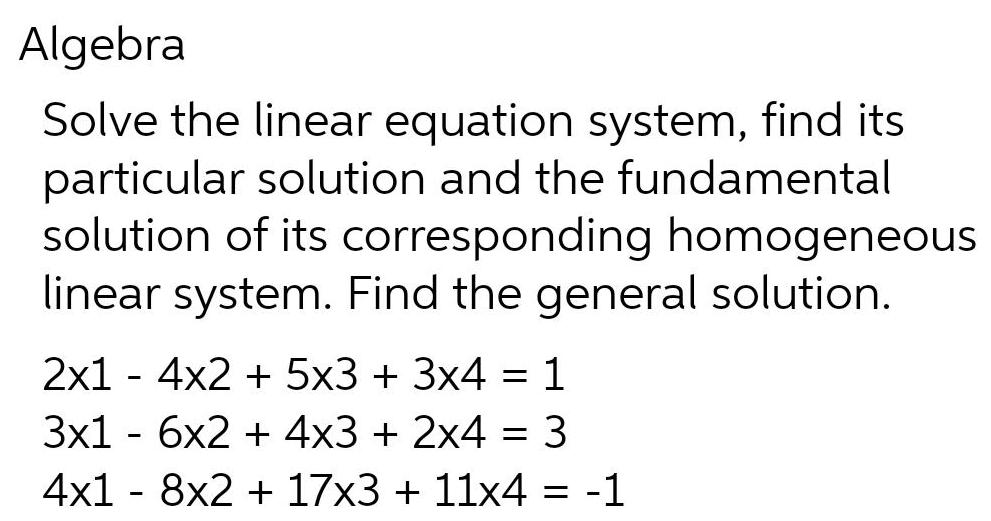Algebra
Matrices & Determinants
Solve the linear equation system, find its particular solution and the fundamental solution of its corresponding homogeneous linear system. Find the general solution. 2x14x2 + 5x3 + 3x4 = 1 3x16x2 + 4x3 + 2x4 = 3 4x18x2 + 17x3 + 11x4 = -1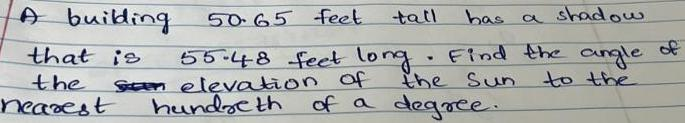Algebra
Matrices & Determinants
A building that is the nearest 50.65 feet. tall has a shadow 55.48 feet long. Find the angle of the Sun to the Sen elevation of hundreth of a degree.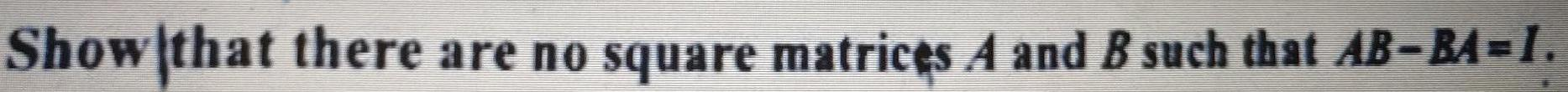Algebra
Matrices & Determinants
Show that there are no square matrices A and B such that AB-BA=1.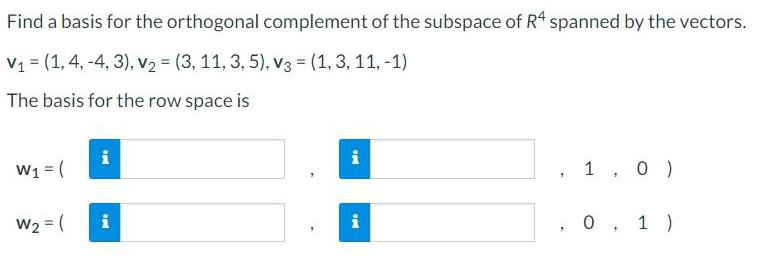Algebra
Matrices & Determinants
Find a basis for the orthogonal complement of the subspace of R4 spanned by the vectors. V₁ = (1, 4, -4, 3), V₂ = (3, 11, 3, 5), V3 = (1, 3, 11, -1) The basis for the row space is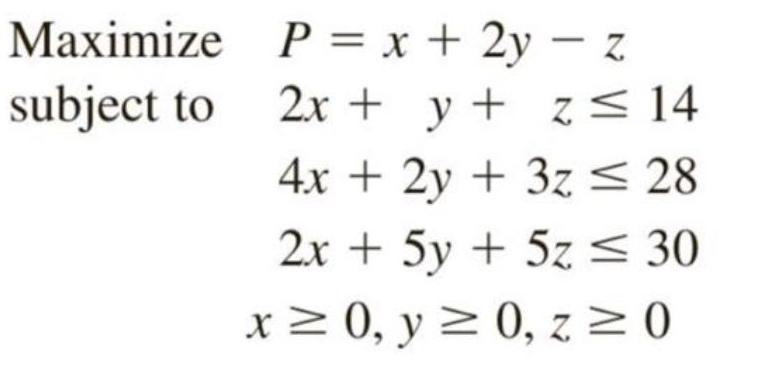Algebra
Matrices & Determinants
Maximize P = x + 2y - z subject to 2x + y + z ≤ 14 4x + 2y + 3z ≤ 28 2x + 5y + 5z ≤ 30 x ≥ 0, y ≥ 0, z ≥ 0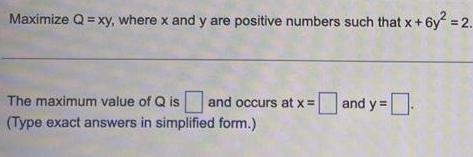Algebra
Matrices & Determinants
Maximize Q = xy, where x and y are positive numbers such that x+6y² = 2.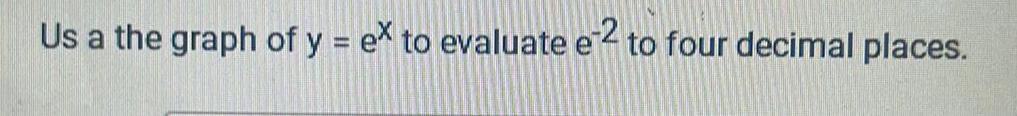Algebra
Matrices & Determinants
Us a the graph of y = ex to evaluate e 2 to four decimal places.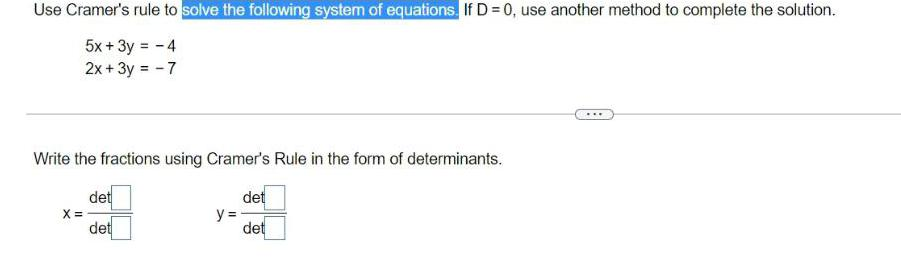Algebra
Matrices & Determinants
Use Cramer's rule to solve the following system of equations. If D = 0, use another method to complete the solution. 5x + 3y = - 4 2x + 3y = -7 Write the fractions using Cramer's Rule in the form of determinants. det det det det X = y = ***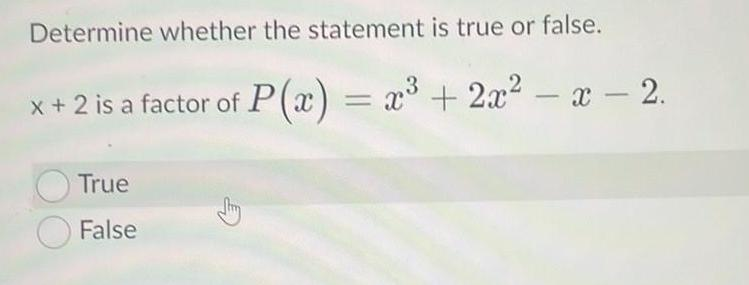Algebra
Matrices & Determinants
Determine whether the statement is true or false. x + 2 is a factor of P(x) = x³ + 2x² - x - 2.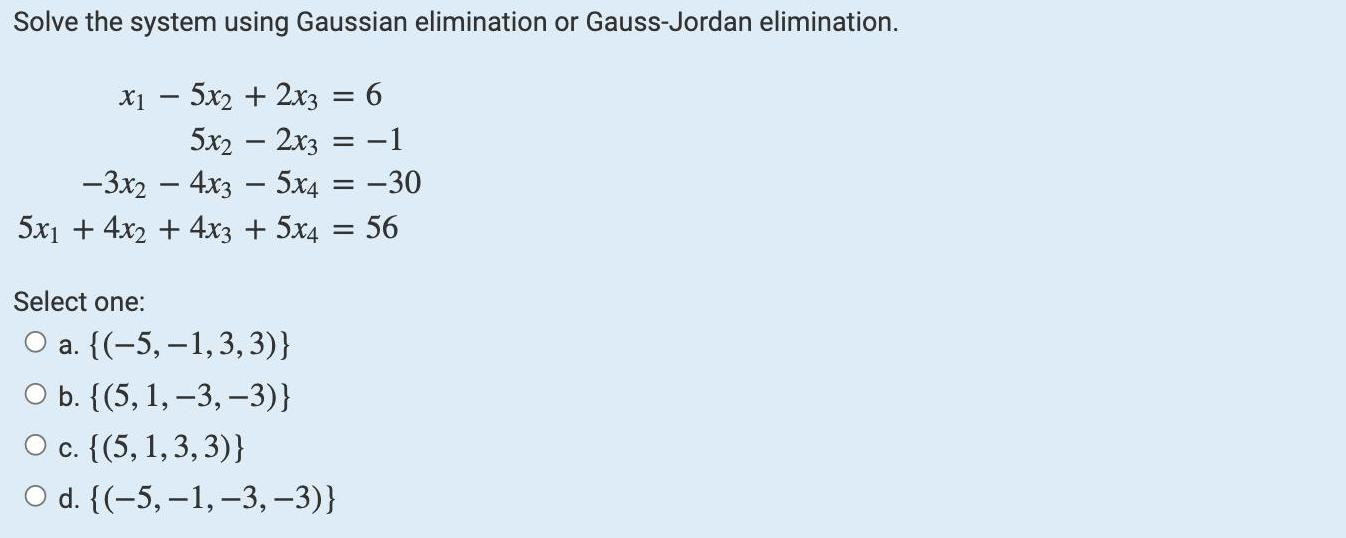Algebra
Matrices & Determinants
Solve the system using Gaussian elimination or Gauss-Jordan elimination. x1- 5x2 + 2x3 = 6 5x2 - 2x3 = -1 -3x2 - 4x3 - 5x4 = -30 5x₁ + 4x2 + 4x3 + 5x4=56 Select one: O a. ((-5, -1,3,3)} O b. {(5, 1, -3, -3)} O c. {(5, 1,3,3)} O d. {(-5, -1, -3, -3)}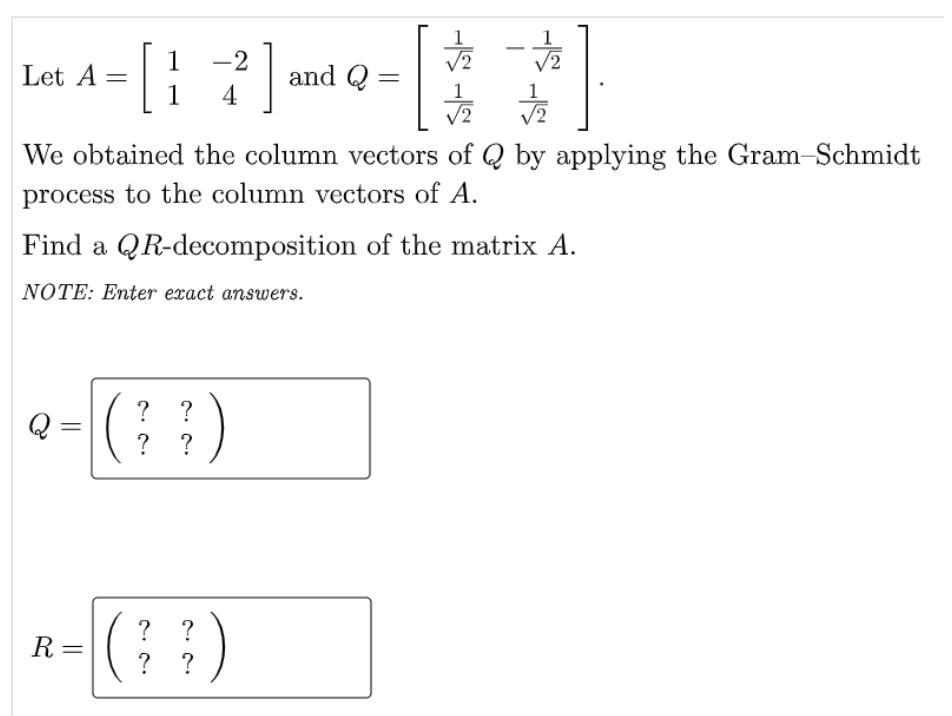Algebra
Matrices & Determinants
Let A = 1 -2 Q = 1/√2 -1/√2 1 4 1/√2 1/√2 We obtained the column vectors of Q by applying the Gram-Schmidt process to the column vectors of A. Find a QR-decomposition of the matrix A. NOTE: Enter exact answers. Q= R=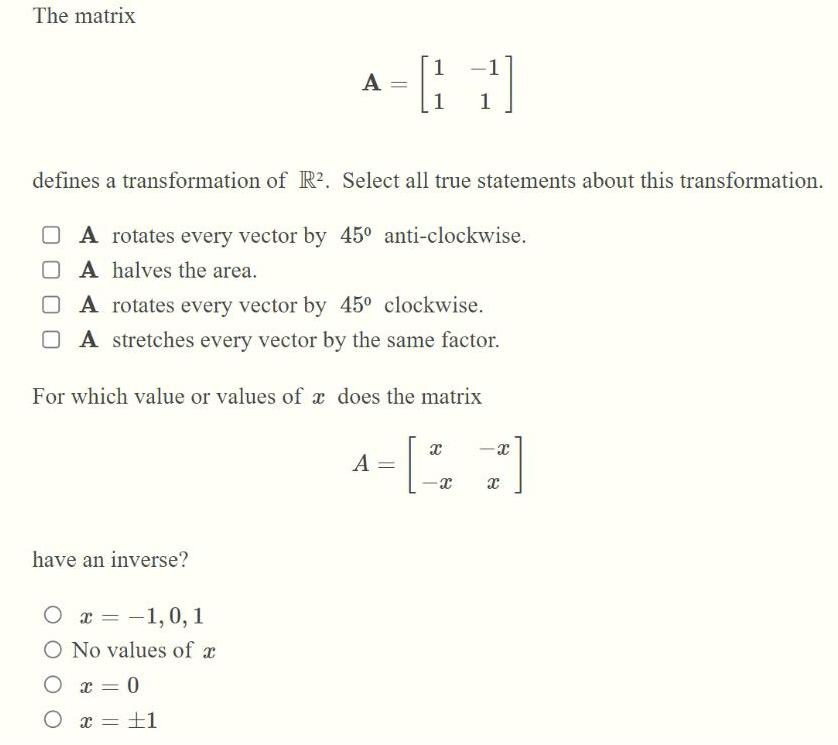Algebra
Matrices & Determinants
The matrix A= 1 -1 1 1 defines a transformation of R². Select all true statements about this transformation. A A rotates every vector by 45° anti-clockwise. A halves the area. have an inverse? A rotates every vector by 45° clockwise. A stretches every vector by the same factor. For which value or values of a does the matrix A= x -x -x x ○ x = -1,0,1 O No values of a O x = 0 O x = = 1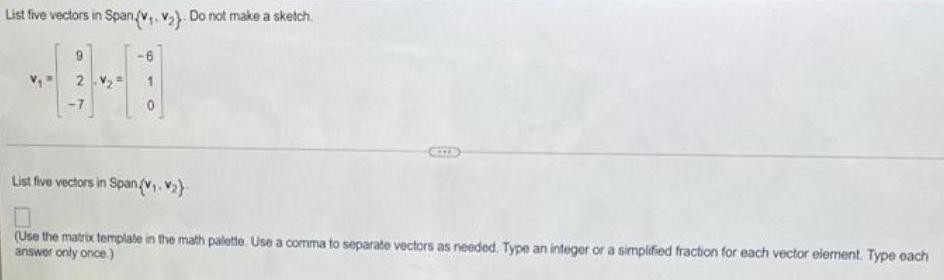Algebra
Matrices & Determinants
List five vectors in Span (V₁ V₂}. Do not make a sketch v1= 9 v2= -6 2 1 -7 0 List five vectors in SpanVv₁.₂} (Use the matrix template in the math palette. Use a comma to separate vectors as needed. Type an integer or a simplified fraction for each vector element. Type each answer only once.)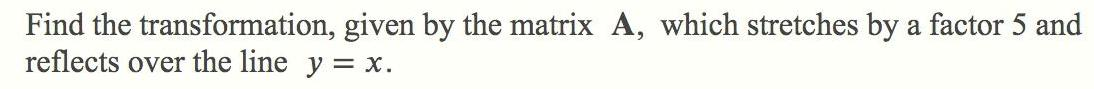Algebra
Matrices & Determinants
Find the transformation, given by the matrix A, which stretches by a factor 5 and reflects over the line y = x.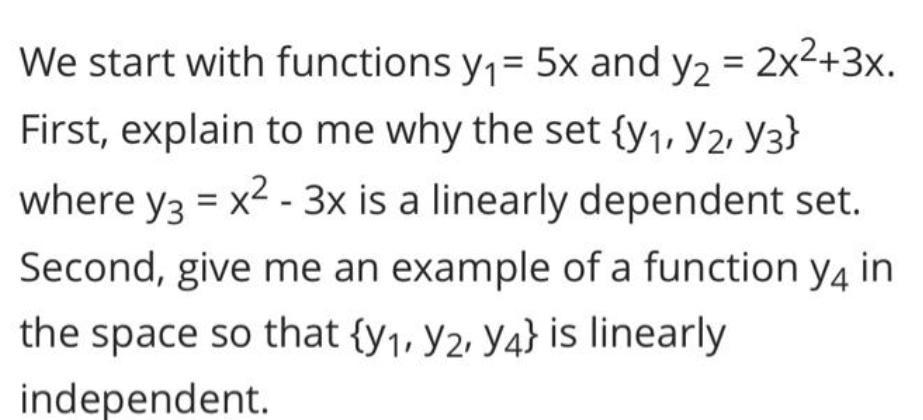Algebra
Matrices & Determinants
We start with functions y₁= 5x and y₂ = 2x²+3x. First, explain to me why the set {y₁, y2, Y3} where y3 = x²-3x is a linearly dependent set. Second, give me an example of a function y4 in the space so that {y₁, y2, Y4} is linearly independent.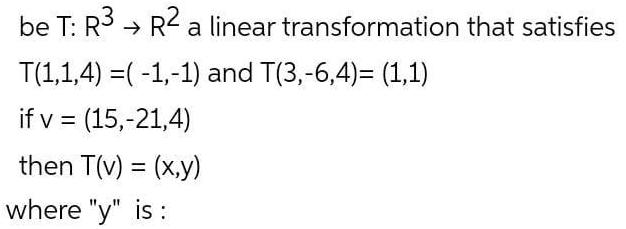Algebra
Matrices & Determinants
be T: R3 R² a linear transformation that satisfies T(1,1,4) =(-1,-1) and T(3,-6,4)= (1,1) if v = (15,-21,4) then T(v) = (x,y) where "y" is: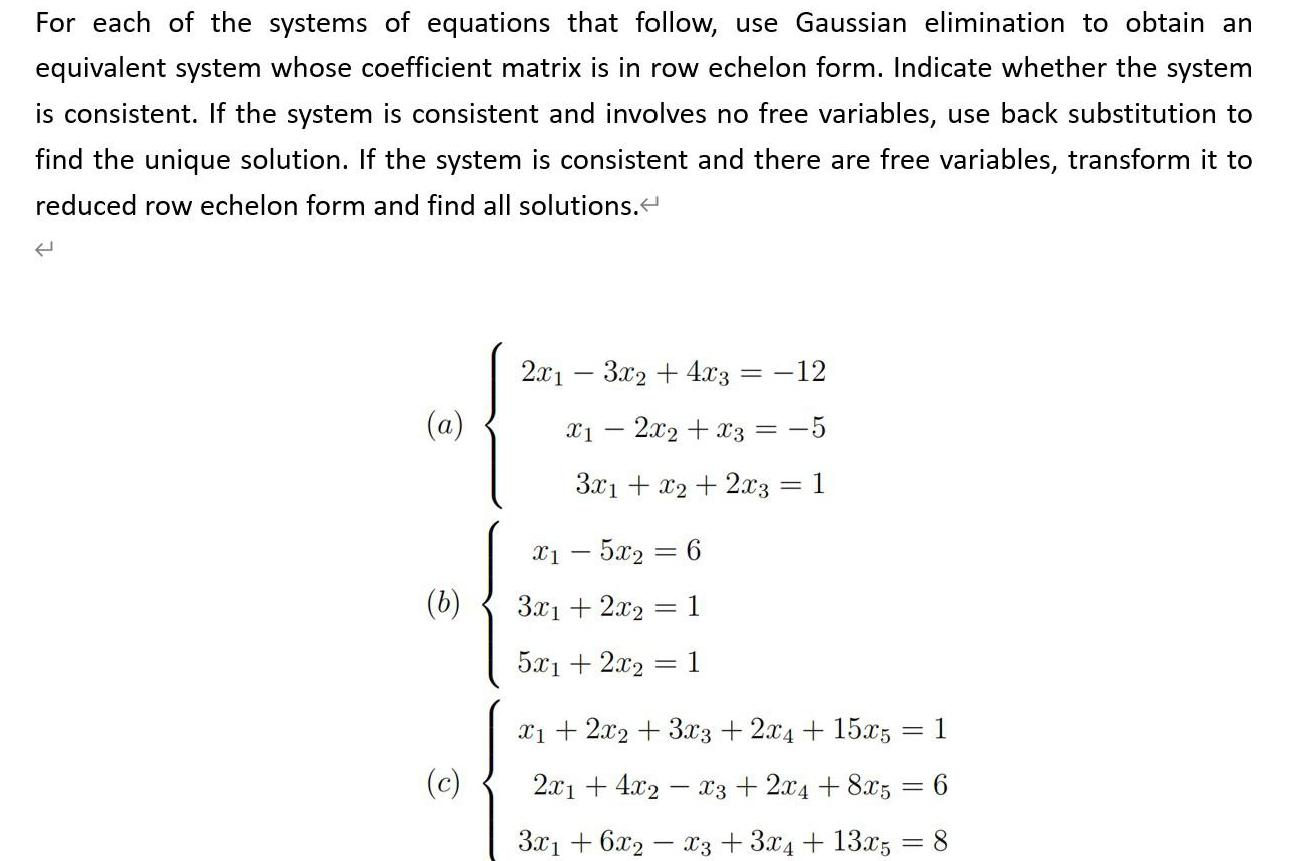Algebra
Matrices & Determinants
For each of the systems of equations that follow, use Gaussian elimination to obtain an equivalent system whose coefficient matrix is in row echelon form. Indicate whether the system is consistent. If the system is consistent and involves no free variables, use back substitution to find the unique solution. If the system is consistent and there are free variables, transform it to reduced row echelon form and find all solutions. (a) 2x1-3x2 + 4x3 = -12 x₁-2x₂ + x3 = -5 3x1 + x2 + 2x3 = 1 (b) x1 - 5x₂ = 6 3x1 + 2x2 = 1 5x1 + 2x₂ = 1 (c) x1 + 2x2 + 3x3 + 2x4 + 15x5=1 2x1+4x2-x3 + 2x4 + 8x5 = 6 3x1+6x2-x3 + 3x4 + 13x5 = 8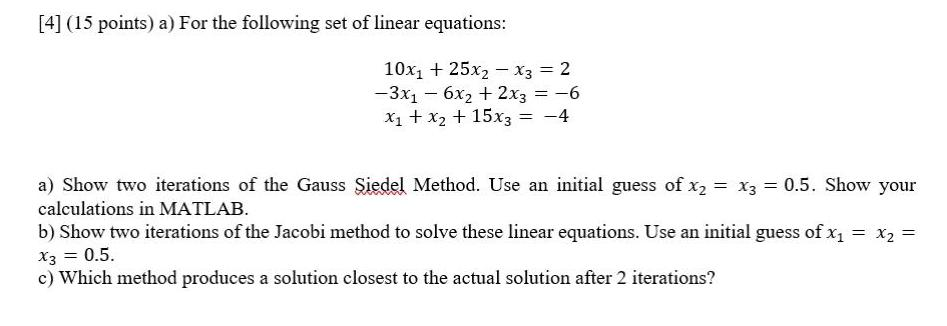Algebra
Matrices & Determinants
a) For the following set of linear equations: 10x₁ + 25x2x3 = 2 -3x₁ - 6x₂ + 2x3 = -6 x₁ + x2 + 15x3 = -4 a) Show two iterations of the Gauss Siedel Method. Use an initial guess of x₂ = x3 = 0.5. Show your calculations in MATLAB. b) Show two iterations of the Jacobi method to solve these linear equations. Use an initial guess of x₁ = x₂ = X3 = 0.5. c) Which method produces a solution closest to the actual solution after 2 iterations?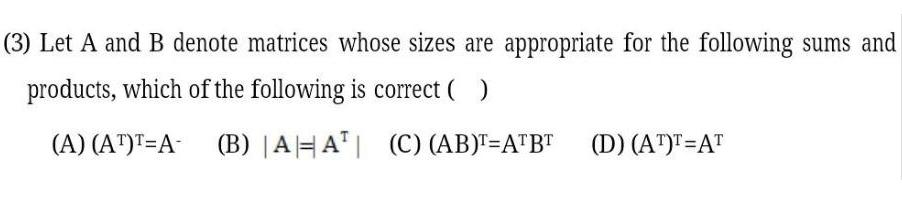Algebra
Matrices & Determinants
Let A and B denote matrices whose sizes are appropriate for the following sums and products, which of the following is correct ( ) (A) (AT)T=A- (B) |A|=|AT| (C) (AB)T=ATBT (D) (AT)T=AT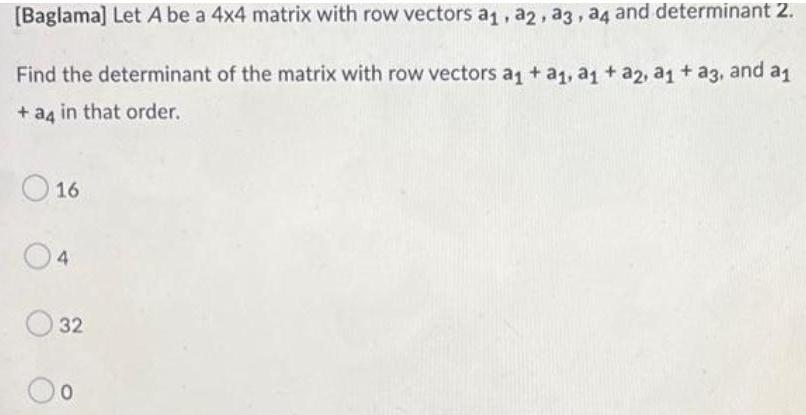Algebra
Matrices & Determinants
Let A be a 4x4 matrix with row vectors a₁, a2, a3, a4 and determinant 2. Find the determinant of the matrix with row vectors a₁ + a₁, a₁ + a2, a₁ + a3, and a₁ + a4 in that order. 16 4 32 0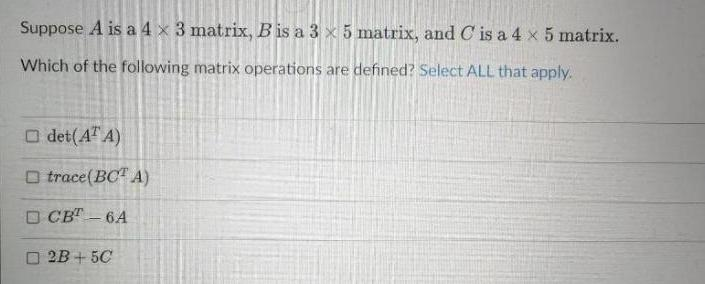Algebra
Matrices & Determinants
Suppose A is a 4 x 3 matrix, B is a 3 x 5 matrix, and C is a 4 x 5 matrix. Which of the following matrix operations are defined? Select ALL that apply. det(ATA) trace (BCTA) CB-T6A 2B+5C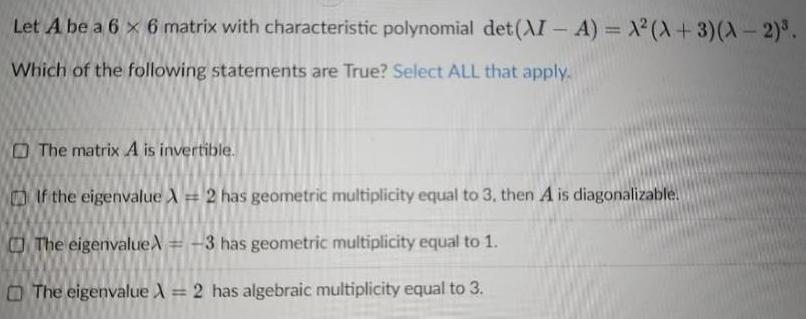Algebra
Matrices & Determinants
Let A be a 6 x 6 matrix with characteristic polynomial det (λI - A) = λ²(λ+3)(λ-2)³. Which of the following statements are True? Select ALL that apply. The matrix λ is invertible. If the eigenvalue λ = 2 has geometric multiplicity equal to 3, then λ is diagonalizable. The eigenvalue λ = -3 has geometric multiplicity equal to 1. The eigenvalue λ = 2 has algebraic multiplicity equal to 3.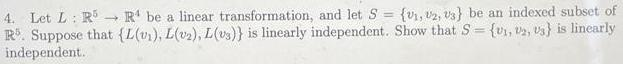Algebra
Matrices & Determinants
→ 4. Let L: R5 R¹ be a linear transformation, and let S = (v₁, 2, 3} be an indexed subset of R. Suppose that {L(v₁), L(v₂), L(vs)} is linearly independent. Show that S= {₁, 2, 3} is linearly independent.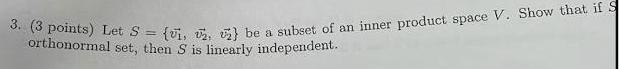Algebra
Matrices & Determinants
Let S = {v1, v2, v3} be a subset of an inner product space V. Show that if S orthonormal set, then S is linearly independent.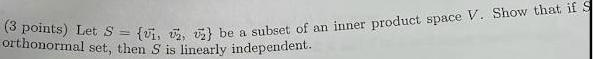Algebra
Matrices & Determinants
(3 points) Let S = {₁, 2, 2 be a subset of an inner product space V. Show that if S orthonormal set, then S is linearly independent.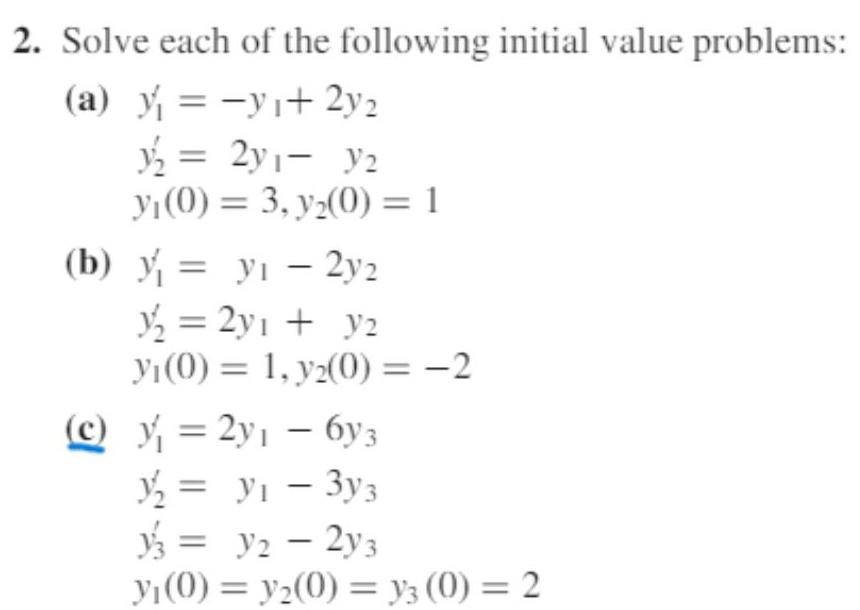Algebra
Matrices & Determinants
2. Solve each of the following initial value problems: (a) y=-y₁+ 2y₂ y/₂ = 2y₁ - y₂ yı(0) = 3, y₂(0) = 1 (b) y yi-2y2 = y₂ = 2y₁ + y₂ yı(0) = 1, y2(0)=-2 (c) y = 2y₁ - 6y3 1/₂ = y₁ - 3y3 = y2 - 2y3 yı(0) = y₂(0) = y3 (0) = 2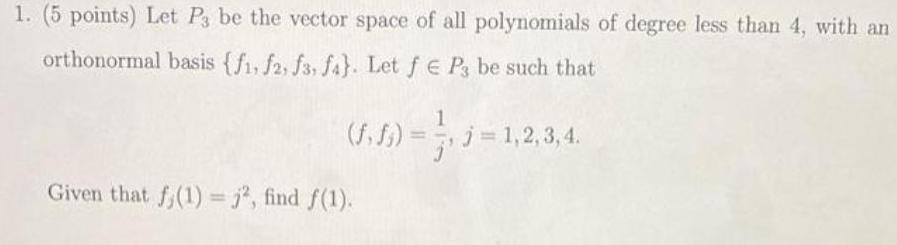Algebra
Matrices & Determinants
1. (5 points) Let P3 be the vector space of all polynomials of degree less than 4, with an orthonormal basis {f1, f2, f3, fa}. Let f € P3 be such that (f.f₁)= Given that f(1) =j², find f(1). 1 , j = 1,2,3,4.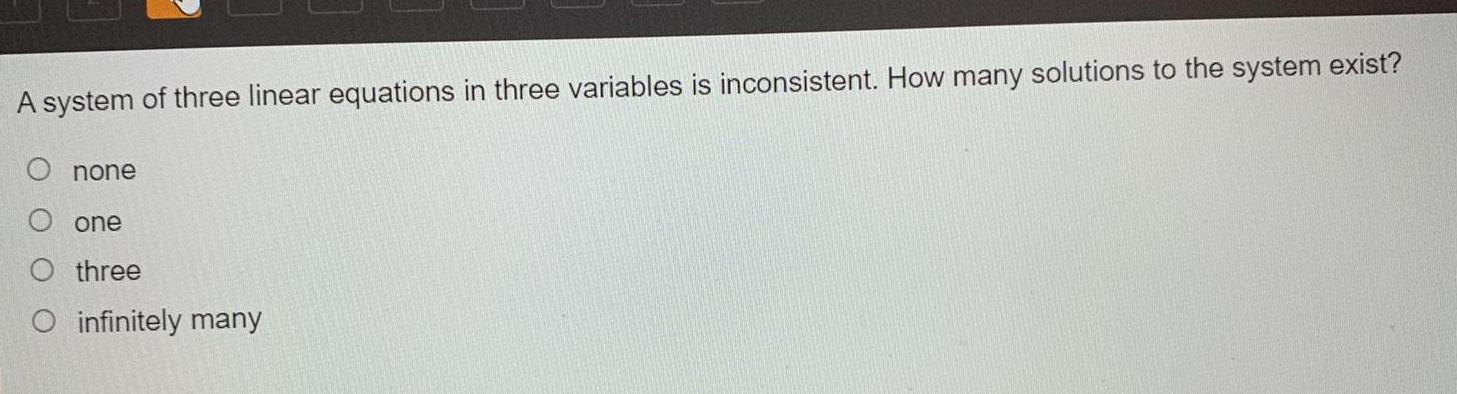Algebra
Matrices & Determinants
A system of three linear equations in three variables is inconsistent. How many solutions to the system exist? O none O one O three O infinitely many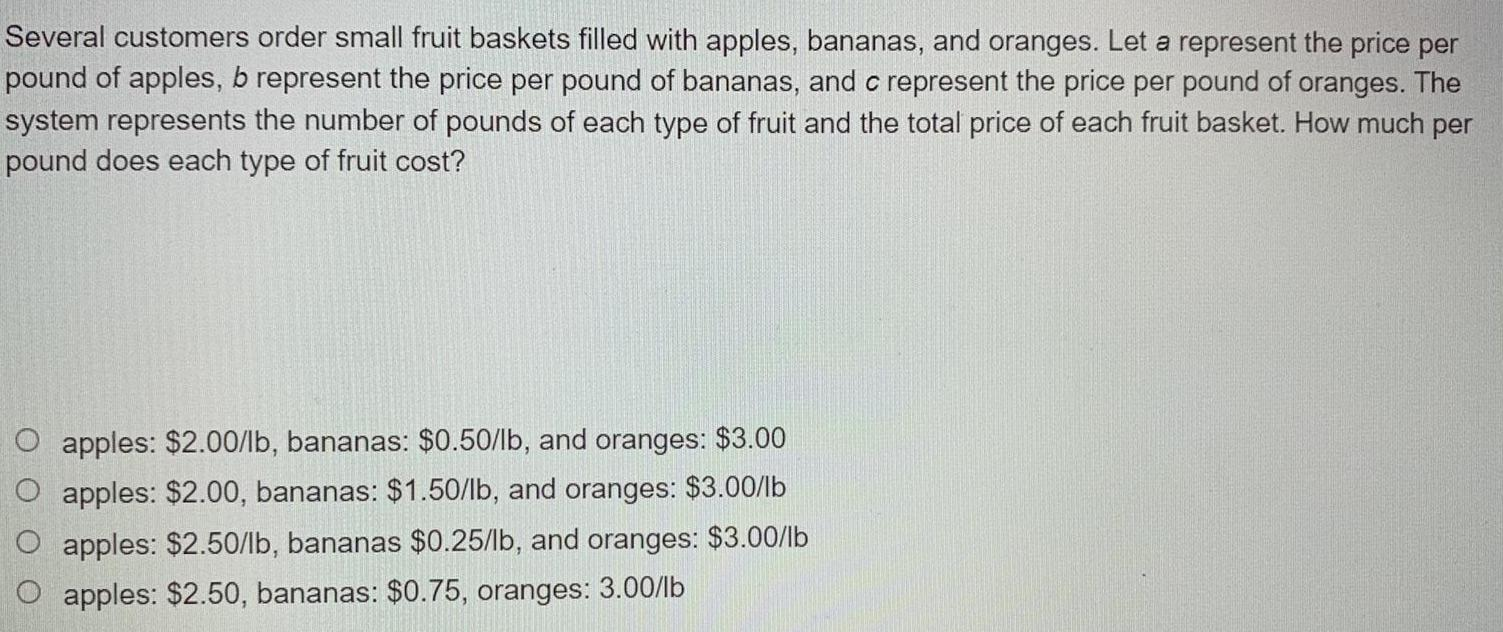Algebra
Matrices & Determinants
Several customers order small fruit baskets filled with apples, bananas, and oranges. Let a represent the price per pound of apples, b represent the price per pound of bananas, and c represent the price per pound of oranges. The system represents the number of pounds of each type of fruit and the total price of each fruit basket. How much per pound does each type of fruit cost? O apples: \$2.00/lb, bananas: \$0.50/lb, and oranges: \$3.00 O apples: \$2.00, bananas: \$1.50/lb, and oranges: \$3.00/lb O apples: \$2.50/lb, bananas \$0.25/lb, and oranges: \$3.00/lb O apples: \$2.50, bananas: \$0.75, oranges: 3.00/lb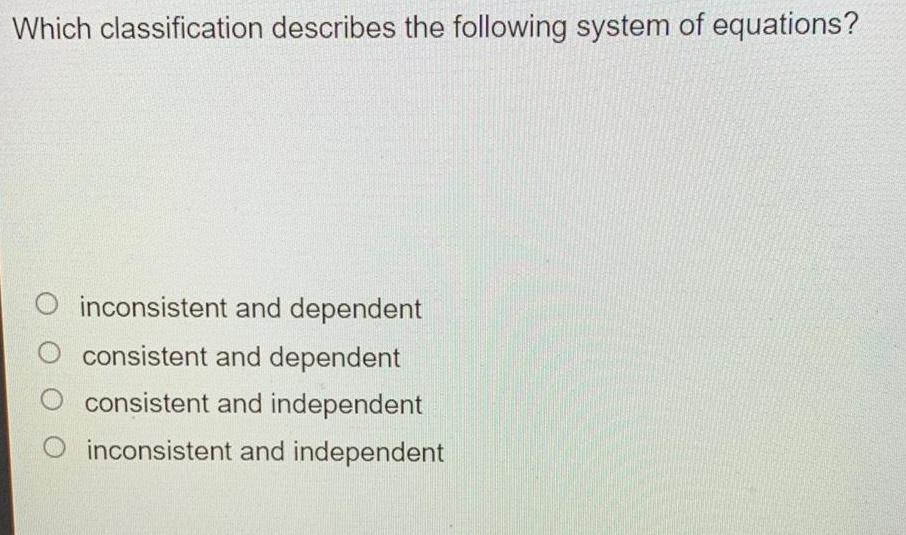Algebra
Matrices & Determinants
Which classification describes the following system of equations? inconsistent and dependent O consistent and dependent consistent and independent O inconsistent and independent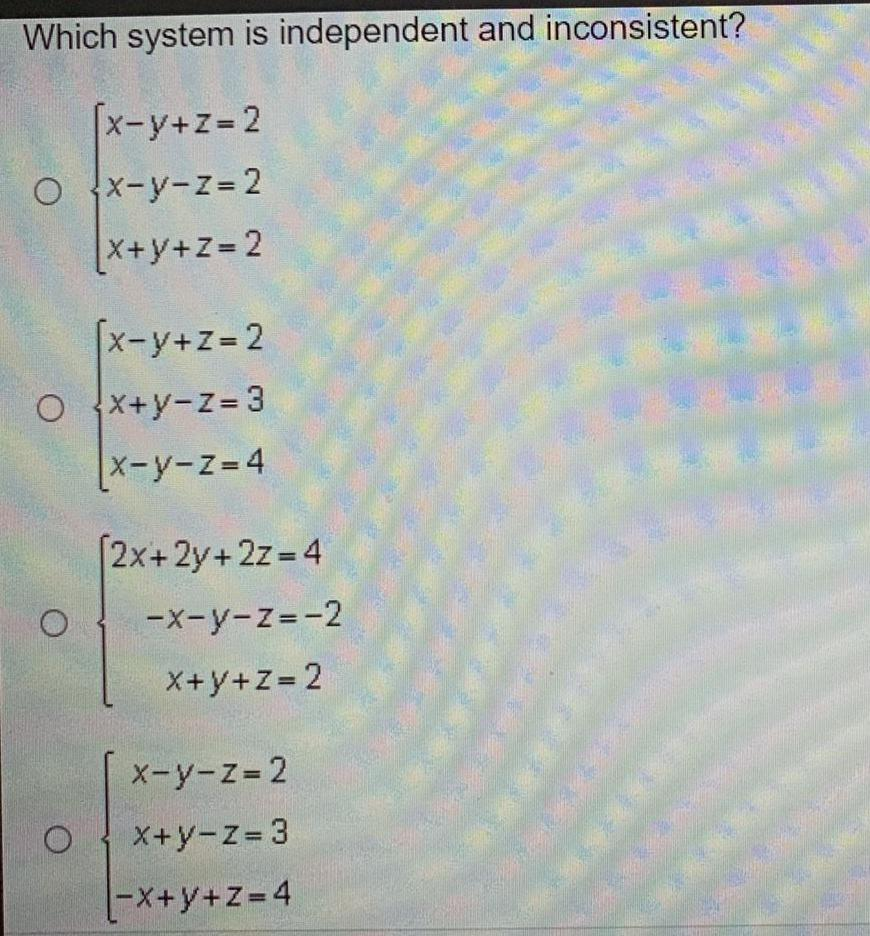Algebra
Matrices & Determinants
Which system is independent and inconsistent? [x-y+z=2 O x-y-z = 2 x+y+z=2 x-y+z=2 Ox+y-z=3 |x-y-z=4 O O [2x+2y+2z=4 -x-y-z=-2 x+y+z=2 x-y-z=2 x+y-z=3 |-x+y+z=4 M tal